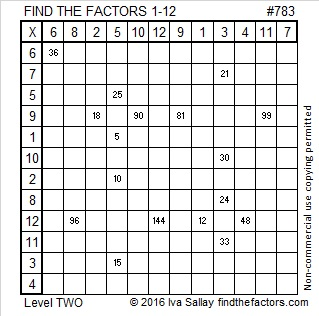# 783 and Level 2

• 783 is a composite number.
• Prime factorization: 783 = 3 x 3 x 3 x 29, which can be written 783 = (3^3) x 29
• The exponents in the prime factorization are 3 and 1. Adding one to each and multiplying we get (3 + 1)(1 + 1) = 4 x 2 = 8. Therefore 783 has exactly 8 factors.
• Factors of 783: 1, 3, 9, 27, 29, 87, 261, 783
• Factor pairs: 783 = 1 x 783, 3 x 261, 9 x 87, or 27 x 29
• Taking the factor pair with the largest square number factor, we get √783 = (√9)(√87) = 3√87 ≈ 27.982137.Here’s today’s puzzle. It’s a level 2 so it isn’t very difficult:Print the puzzles or type the solution on this excel file: 12-factors-782-787

—————————————

27 x 29 = 783. Since (n – 1)(n + 1) always equals n² – 1, we know that 783 is one number away from the next perfect square.

29 is a factor of 783, making 783 the hypotenuse of a Pythagorean triple:

• 540-567-783, which is 27 times 20-21-29.
• Thus 540² + 567² = 783² just as 20² + 21² = 29².

783 is also a palindrome in bases 15, 23, and 28:

• 373 BASE 15; note that 3(225) + 7(15) + 3(1) = 783
• 1B1 BASE 23 (B is 11 base 10); note that 1(23²) + 11(23) + 1(1) = 783
• RR BASE 28 (R is 27 base 10); note that 27(28) + 27 = 783

—————————————This site uses Akismet to reduce spam. Learn how your comment data is processed.